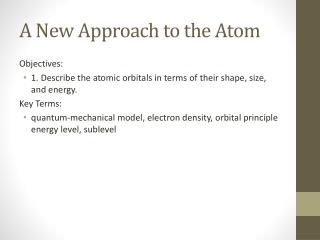DownloadDownload PresentationA New Approach to the Atom

# A New Approach to the Atom

Download Presentation## A New Approach to the Atom

- - - - - - - - - - - - - - - - - - - - - - - - - - - E N D - - - - - - - - - - - - - - - - - - - - - - - - - - -
##### Presentation Transcript

1. A New Approach to the Atom Objectives: • 1. Describe the atomic orbitals in terms of their shape, size, and energy. Key Terms: • quantum-mechanical model, electron density, orbital principle energy level, sublevel

2. Probability & Orbitals Schrodinger stated that electron orbitals can more correctly referred to as electron clouds. • An orbital is the region around the nucleus where an electron is likely to be found. • Within an orbital it is more likely to find the electron nearer to the nucleus than toward its boundary • There are 4 basic orbital types: s,p,d,& f orbitals. • Each orbital can hold a maximum of 2 electrons each with opposite spin

3. s orbital • Spherical in shape • 1st orbital in any energy level and only orbital located at n=1

4. p orbital • The p orbital is dumbbell shaped • The p-orbitals begins in the 2nd energy level • The are 3 orbitals in the p-orbital system • The orbitals extend out on the x, y, & z axis (px,py,pz) • The system can hold a maximum of 6 electrons

5. d-orbital • The d orbital is dumbbell and donut shaped • The d-orbital begins in the 3rd energy level • The are 5 orbitals in the d-orbital system • The orbitals extend out at angles to the x, y, & z axis • The system can hold a maximum of 10 electrons • Considered a suborbital system and is inserted after 4s

6. f-orbital • The f-orbitals begins in the 4th energy level • The are 7 orbitals in the d-orbital system • The orbitals extend out at angles to the x, y, & z axis • The system can hold a maximum of 14 electrons • Considered a suborbital system to the d-block and is inserted after 6s

7. Orbital Summary for the Principle Energy Levels • The number of the energy level is equal to the number of orbital sublevels • n=1 1 sublevel 1s (2 electrons) • n=2 2 sublevels 2s, 2p (8 electrons) • n=3 3 sublevels 3s, 3p, 3d (18 electrons) • n=4 4 sublevels 4s, 4p, 4d, 4f (32 electrons) Number of electrons possible for each orbital sublevel: • s = 1 (2 electrons) • p = 3 (6 electrons) • d = 5 (10 electrons) • f = 7 (14 electrons)